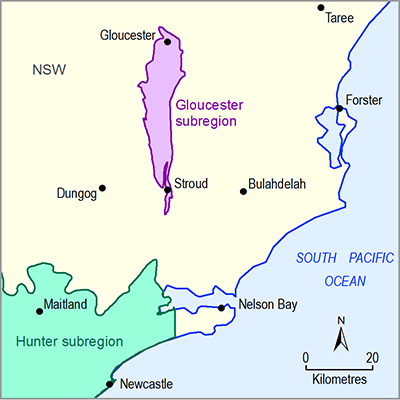# 2.6.2.3.4 Model code and solver

Page 17 of 60

### 2.6.2.3.4.1Analytic element model

For this groundwater model the analytic element methodology was selected (Bakker, 2013) using the open-source implementation available in TTim (Bakker, 2015). The groundwater flow equations were solved based on the representation of internal boundary conditions, points, lines or polygons, where constant groundwater level, constant flux or flux dependence on groundwater level is imposed. By superposing these flow equations, groundwater level and flux can be evaluated at arbitrary points in space and time. The solution is therefore independent of a spatial and temporal discretisation of the model domain and time into grid and time steps.

The groundwater model was created as a Python script and solved using a 64-bit Python installation and the 64-bit distribution of the TTim package. The script, together with the input files and documentation, are available at http://www.bioregionalassessments.gov.au.

### 2.6.2.3.4.2Alluvial MODFLOW models

For this groundwater model the industry standard MODFLOW model is used (Harbaugh et al., 2000), MF2000 1.18 version (USGS, 2014). The groundwater flow equations are solved based on a finite-difference solution of the three-dimensional groundwater flow equation, in a time-stepping manner where the previous solutions for groundwater heads and fluxes are used as the initial conditions for the next time period.

The time requirement for a solution of the equations is most often driven by the number of grid cells and layers. The fact that the alluvium is relatively sparse (see Figure 10, only about 10% of the model area is active alluvium cells) makes the computational effort small, requiring about 30 seconds for 100 years of simulated heads. The main driver of actual run time, however, is the amount of time spent reading and writing data to disk storage, sometimes over a network. For this reason, the fewest possible number of files are written and stored, fast temporary storage is used where available, and larger files required for water balance summary and head observation are deleted after analysis of each run. The model files and executables, including the technical information about running the model, are available at http://www.bioregionalassessments.gov.au.

Last updated:
5 November 2018